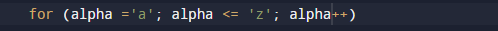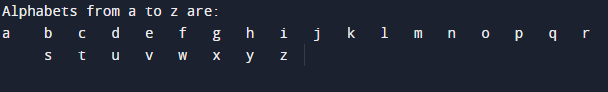C Program to Print Alphabets from a-z(small)

In this tutorial you will learn about the C Program to Print Alphabets from a-z(small) and its application with practical example.

In this tutorial, we will learn to create a C program which will print alphabet  form a-z(Small)  using C programming.

Prerequisites

Before starting with this tutorial we assume that you are best aware of the following C programming topics:

• c data type
• C  loops.
• Basic input/output.

C Program to Print Alphabets from a-z(small)

In the following  program with the help of for loop we will print alphabet form ‘a-z‘. Firstly we take a variable name ‘alpha‘  of type character .Then initialize loop form alpha=’a’ that will goes till alpha=’z’  and the structure of loop be like thiswithin the loop body print the value of  alpha.

C Program to Print Alphabets from a-z(small).

In this program we will print value from a -z using for loop.
Firstly we declare required header file and variable. Next we print complete value form a-z using or loop.
Let’s take a  have look.

OutputIn the above program, we have first declared and initialized a set variables required in the program.

• alpha= for holding value from a-z.

Here we creating a program in which we print series from ‘a-z’

Here first we take a variable name alpha and Initialize loop  variable from alpha=’a’, that goes till alpha=’z’ , then increment the loop each iteration by 1. The structure of loop look like thiswithin the loop we print the value of alpha and we will get the following series.In this program, the loop display the English alphabet in lowercase.

In this tutorial we have learn about the C Program to Print Alphabets from a-z(small) and its application with practical example. I hope you will like this tutorial.